## Basics of Cells & Battries

• Primary cell
• Secondary cell
• Reserve cell
• Fuel cell

Primary cell:

1. primary cell or battery is one that cannot easily be recharged back.
2. Generally these cells contain electrolyte in the form of solid powdered state and that’s why these are also known as DRY CELLS.
3. Cells contain electrochemical reactions which are non –reversible.
4. These are having very low self discharge rate known as charge retention factor. Factors affecting self-discharge extent include: anode and cathode preparation course, electrolyte nature and concentration, and battery storage temperature and time, among which battery self discharge greatly depends on temperature.
5. Examples: Zn–MnO2 cell which is most commonly used battery. This type of battery is available in different sizes- AA, AAA, C, D etc.

We discuss later on what is the difference in between them in terms of electrochemistry.

Let us understand electrochemistry of Zn –MnO2 cell: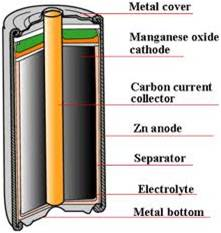Understanding electrochemistry of any battery always divides the cell into separate anode region, cathode region, electrolyte used and separator material which separate anode and cathode.

So, here we have Zn metal at anode which reacts with electrolyte ammonium chloride as follows:

Zn(s) + 2OH(aq) → ZnO(s) + H2O(l) + 2e [e° = 0.76 V]

Emf(Ox) = 0.76 – RT/2F ln([Zn0]/[OH-]^2)……nernst equation

At  cathode MnO2 is there which will reat with two e-‘s on cathode eletrode surface i.e.

2MnO2(s) + H2O(l) + 2e → Mn2O3(s) + 2OH(aq) [e° = +0.5 V]

Emf (Red) = 0.5 – RT/2F ln ([Mn2O3]/[MnO2^2])

Add both anodic and cathodic half cell reactions we get:

Zn +MnO2 –>ZnO2 + Mn2O3

Emf total = Emf (ox) + Emf (red)

= +1.56 –RT/2F ([Mn2O3][ZnO2]/[MnO2])

The above process is discharge of cell but if we consider discharge of a battery we have to reverse the above reactions but thermodynamically this process is infeasible, and that’s why we cannot charge primary cells.

Secondary cell:

1. Electrochemical reaction occurring in reversible in nature ,therefore we can recharge them
2. e.g. : lead–acidnickel cadmium (NiCd), nickel metal hydride (NiMH), lithium ion(Li-ion), and lithium ion polymer (Li-ion polymer).

Lead–acid batteries, invented in 1859 by French physicist Gaston Plante, are the oldest type of rechargeable batteries. This is most widely used commercial battery used in    automobiles and heavy electronic devices because of its high current rating.

Let’s understand the mechanism of lead acid batteries:

Negative plate reaction: Pb(s) + HSO−4(aq) → PbSO4(s) + H+(aq) + 2e-

Positive plate reaction: PbO2(s) + HSO−4(aq) + 3H+(aq) + 2-e → PbSO4(s) + 2H2O(l)

Discharging–>

Overall reaction: Pb(s) + PbO2(s) + 2H2SO4(aq) → 2PbSO4(s) + 2H2O(l)

<–charging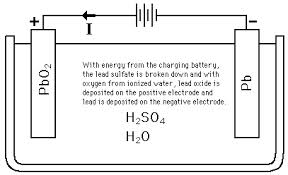Applying Nernst equation at room temperature for this case we get 2v.

I.e. Per cell voltage is 2v.Therefore 6 cells are stacked together to form 12v battery having 13.9 amp-hours per kg.

Similarly we can think of Lithium ion battery, they are one of the most popular types of rechargeable battery for portable electronics, with one of the best energy densities, and only a slow loss of charge when not in use.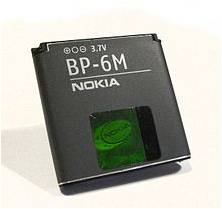Reactions:
Positive electrodes: LiCoO2- =   Li1-x CoO2 + xLi + xe-
Negative electrodes:  C+xLi+xe-  =   CLix
Discharging–>

Battery as whole:    LiCoO2 + C  =   Li1-x CoO2+CLix
<– charging

Now next is Reserve batteries :

•  These batteries are working as normal batteries but cell is inactive (dead) until and unless cell certain condition is not fulfilled.
•  These batteries are used in radio sondes, missiles, projectile and bomb fuzes, and various weapon systems. Generally these are used for military purposes.
•  Another example is zinc-air batteries where the cell is sealed until use: a tab is removed to admit air and activate the cell.
•  It can be activated by: Addition of water, Addition of electrolyte, Introducing gas into the cell, heating solid electrolyte (solid-oxide fuel cell) so that it becomes conductive and there may be many other ways by which we can activate these cells.

Fuel cell

fuel cell is a device that converts the chemical energy from a fuel into electricity through a chemical reaction with oxygen or another oxidizing agent.

Hydrogen is the most common fuel, but hydrocarbons such as natural gas and alcohols like methanol are sometimes used.

Fuel cells are different from batteries in that they require a constant source of fuel and oxygen to run, but they can produce electricity continually for as long as these inputs are supplied. For e.g. in Zn-Air fuel system if continuous supply of O2 at cathode and simultaneously removal of ZnO (by product) from anode we can get power continuously.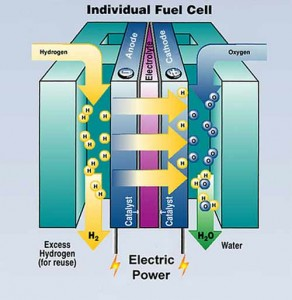For e.g.- Hydrogen fuel cell, Microbial fuel cell etc.

So, until now we have discussed types of batteries, let us now understand basic things which very essential to understand any battery :

Terminology used in Electrochemistry:

1) Ampere hour: It is the amount of current supplied by the battery in one hour.

e.g.: 30mAhr means when connected to a load of rating 5 mAhr then load will work for 6 hrs .When cells are stacked together then ampere hr rating is return of per kg of cell.

2) Energy Density:  It is how much energy can be stored per unit volume or mass of a battery. It is expressed in joules per unit mass. MJ/kg

3) Power Density: It is basically how quickly energy is taken out from battery.It is expressed in kW/kg

4) Self discharge rate: it is process in which cell stored internal energy is decreased. self- discharge in a battery occurs is dependent on the type of battery, state of charge, charging current, ambient temperature and other factors.

Among rechargeable batteries, lithium batteries suffer the least amount of self-discharge (around 2–3% discharge per month), while nickel-based batteries are more seriously affected by the phenomenon (nickel cadmium, 15–20% per month; nickel metal hydride, 30% per month).

Now let us understand on what factors cell voltage, internal resistance depends:

Cell Parameters

1)     CELL VOLTAGE : it depends upon concentration of chemicals.

• E call= Rt/nF*ln[k]….Nernst equation
• Where k depends upon concentration of reactants and products. Now when cell discharges reaction will go in forward direction till equilibrium is reached.(le chatlier principle).Then cell is considered as dead. Now we have to recharge the cell to increase [] of product side.

For e.g. we have AA and AAA type of dry cell, so what is the difference in between them???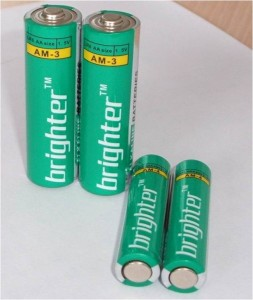• Diameter of AA battery is more AAA battery.
• Both are having same voltage output as voltage output does not depends upon size of cell but depends upon concentration of electrolytes.
• The only difference is of current rating which is less in AAA batteries as compared to AA. e.g.: AA, AAA, LITHIUM BATTERY, ALKALINE BATTERIES ETC.(’AA ’ means 50.5mm x 13.5mm.)

2)     Internal Resistence

•   Resistance =ρ *l/a where l is length between electrodes and ‘a’ is surface area of electrodes.
•  Now this constant ρ depends upon electrolyte concentration. In 90% batteries electrolyte work as catalyst but in few cells electrolyte is consumed .As electrolyte becomes less and less conductive .so result is increased resistivity of cell.

3)     Polarization curve: It is plot between voltage outputs of the battery versus % discharged.

Figure shown here is the polarization curve for most commonly used batteries. We can see here initial voltage drop then it remains constant and then finally voltage drops fast.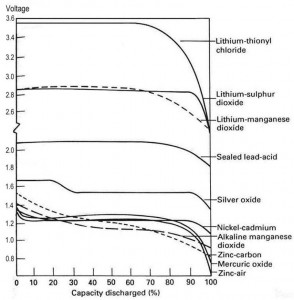Other interesting stuffs:

The battery terminals are generally labeled as : + , – , T , and D or Do.
T is connected to an internal temperature sensitive component ( thermistor) . This is to prevent overheating of the battery so it is very important on safety point of view
D or Do is a newly introduced contact. It is a data line which allows Smartphone and battery to exchange information about current, voltage, temperature, residual battery capacity etc…. It has been introduced to improve % remaining battery capacity readings.

CEV - Handout